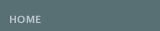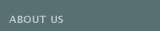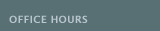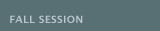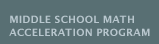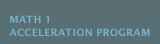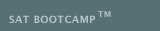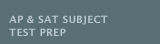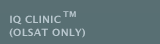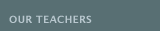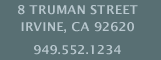MATH 7 ACCELERATED (MSMAP) is designed for the end of course (EOC) test for the 7th grade student for MATH 1 placement in the 8th grade. This group class is aligned with the California's COMMON CORE State Standards: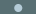Ratios and Proportional Relationships: Use proportional relationships to solve multistep ratio and percent problems.Expressions and Equations: Use variables to represent quantities in a real-world or mathematical problem, and construct equations and inequalities to solve problems by reasoning about the quantities.Geometry: Use facts about supplementary, complementary, vertical, and adjacent angles in a multi-step problem to write and solve equations for an unknown angle in a figure. Solve real-world problems involving area, volume and surface area of 2 and 3 dimensional objects composed of triangles, quadrilaterals, polygons, cubes, and right prisms.Statistics and Probability: Investigate chance processes and develop, use, and evaluate probability models. Develop a probability model and use it to find probabilities of events.
 Students will be required to attend twice a week (one lesson to emphasize problem solving and word problems and the other to reinforce accuracy). Students are expected to complete additional exercise problem sets for homework. CAASPP test preparation will be integrated in the weekly lectures. Students can expect homework, quizzes, a mid-term and a final. Grades will be given.
 All seventh graders must first pass the end of year test in order to take Math 1 Acceleration Test in the Fall.
 M00A Math 7 (Math 1 Placement-MSMAP)
 August 19June 5 (42 weeks)* *November 2530 (Thanksgiving Recess) *December 23January 5 (Winter Recess) *March 30April 5 (Spring Recess)
 Thursdays 4:00-6:00pm KIMES
 December 2April 24 (21 weeks)* *December 23January 5 (Winter Recess) *March 30April 5 (Spring Recess)
 Mondays or Wednesdays 4:00-6:00pm
 MATH 8 (MSMAP) is designed for the end of course (EOC) test for MATH 1 placement the following year. This course introduces the language and methods of algebra. Instruction will focus on 4 critical areas:Expressions and Equations: Solve real-world and mathematical problems of two linear equations in two variables algebraically, and estimate solutions by graphing the equations.Functions: Interpret the equation y=mx+b as defining a linear function. Construct a function to model a linear relationship between two quantities.Geometry: Apply the Pythagorean Theorem to determine unknown side lengths in right triangles in real-world and mathematical problems in two and three dimensions. Apply the formulas for the volumes of cones, cylinders, and spheres and use them to solve real-world and mathematical problems.Statistics and Probability: Construct and interpret scatter plots for bivariate measurement data to investigate patterns of association between two quantities.
 Students will be required to attend twice a week (one lesson to emphasize problem solving and word problems and the other to reinforce accuracy). Students are expected to complete additional exercise problem sets for homework. CAASPP test preparation will be integrated in the weekly lectures. Students can expect homework, quizzes, a mid-term and a final. Grades will be given.
 M01A MATH 8 (Math 1 Placement-MSMAP)
 August 19June 5 (42 weeks)* *September 2 (Labor Day Observed) *October 14 (Columbus Day Observed) *November 2530 (Thanksgiving Recess) *December 23January 5 (Winter Recess) *March 30April 5 (Spring Recess)
 Mondays 4:00-6:00pm FU
 December 2April 24 (21 weeks)* *December 23January 5 (Winter Recess) *March 30April 5 (Spring Recess)
 Wednesdays 4:00-6:00pm